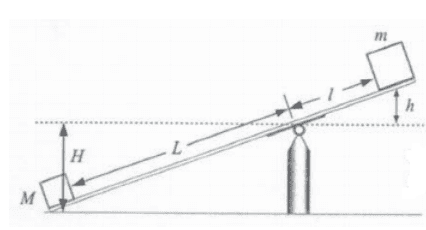# Two blocks and a board

• RubroCP
Then use the equation for change of potential energy: ##\frac{\Delta U_L}{\Delta U_R}=-\frac{MH}{mh}##.

#### RubroCP

Homework Statement
Consider a board like the one in the figure below. Assuming that the potential energy does not change when placing the balance in a horizontal position, and disregarding the mass of the board, show:

a) The relationship between the potential energy gained by the object of mass ##M## and the decrease in the potential energy of the object of mass ##m## when the balance is positioned horizontally.

b) The relationship between the quantities ##M##, ##m##, ##L## and ##l##.
Relevant Equations
There's noneHello, thanks for the attention. Well, knowing that the only acting force is the gravitational force, I stated that ##U=-MgH## for the ##M## mass block and that ##U=mgh## for the ##m## mass block. After that I divide the two and got the relationship for the alternative "a". For alternative "b" I used the equilibrium condition and stated that ##MgL=mgl##, such that ##\frac{M}{m}=\frac{l}{L}##. But I don't know if it's correct. Could you help me with the resolution?

Last edited:
•Delta2
Part (a) asks for a relationship between the two potential energies. You have found each one separately, but you have not provided the required relationship.

How do you know that the system is in equilibrium when the board is horizontal? Can you prove it or are you guessing?

Last edited:
•RubroCP
kuruman said:
Part (a) asks for a relationship between the two potential energies. You have found each one separately, but you have not provided the required relationship.

How do you know that the system is in equilibrium when the board is horizontal? Can you prove it or are you guessing?
Thanks, kuruman. My question is regarding the division in alternative "a". As the blocks move I got a negative and a positive potential. In the division, should I analyze the module or leave the value as negative? For alternative "b", I started from what the statement stated:

"Assuming that the potential energy does not change when placing the balance in a horizontal position [...]"

Would that be enough to say that balance occurs?

•Delta2
You still have not answered part (a). Let
##\Delta U_L## = potential energy change due to the motion of the mass on the left
##\Delta U_R## = potential energy change due to the motion of the mass on the right

Find an equation relating the two, e.g. ##\Delta U_L=\frac{\pi}{2}\Delta U_R## or something like that.

RubroCP said:
Would that be enough to say that balance occurs?
Why would it be? Convince me with equations or with some logical reasoning that balance occurs. If you do part (a), it will be easier to see what's going on.

kuruman said:
You still have not answered part (a). Let
##\Delta U_L## = potential energy change due to the motion of the mass on the left
##\Delta U_R## = potential energy change due to the motion of the mass on the right

Find an equation relating the two, e.g. ##\Delta U_L=\frac{\pi}{2}\Delta U_R## or something like that.

Why would it be? Convince me with equations or with some logical reasoning that balance occurs. If you do part (a), it will be easier to see what's going on.
Well, I could affirm that ##\frac{\Delta U_L}{\Delta U_R}=-\frac{MH}{mh}##. But, knowing that ##\frac{H}{h}=\frac{L}{l}##, then ##\frac{\Delta U_L}{\Delta U_R}=-\frac{ML}{ml}##. But, how can I prove that ##\Delta U_L=\Delta U_R## in this case?

RubroCP said:
Well, I could affirm that ##\frac{\Delta U_L}{\Delta U_R}=-\frac{MH}{mh}##. But, knowing that ##\frac{H}{h}=\frac{L}{l}##, then ##\frac{\Delta U_L}{\Delta U_R}=-\frac{ML}{ml}##.
Affirm? The statement of the problem has already told you something in the statement "Assuming that the potential energy does not change when placing the balance in a horizontal position, ##\dots~##Can you express that with an equation? What potential energy is it referring to? How would you cast this assumption into a mathematical equation?
RubroCP said:
But, how can I prove that ##\Delta U_L=\Delta U_R## in this case?
You cannot because it isn't true.

RubroCP said:
But, how can I prove that ΔUL=ΔUR in this case?
I think the correct is ##\Delta U_L=-\Delta U_R##. To prove it start from the given fact from the statement of the problem, that the potential energy of the system remains constant in those two positions.